# 【C进阶】 字符串函数和字符分类函数

 阿里云国际，腾讯云国际，AWS 免费开户实名账号 代冲值 优惠多多 微信号：monov8 飞机：@monov8

# 前言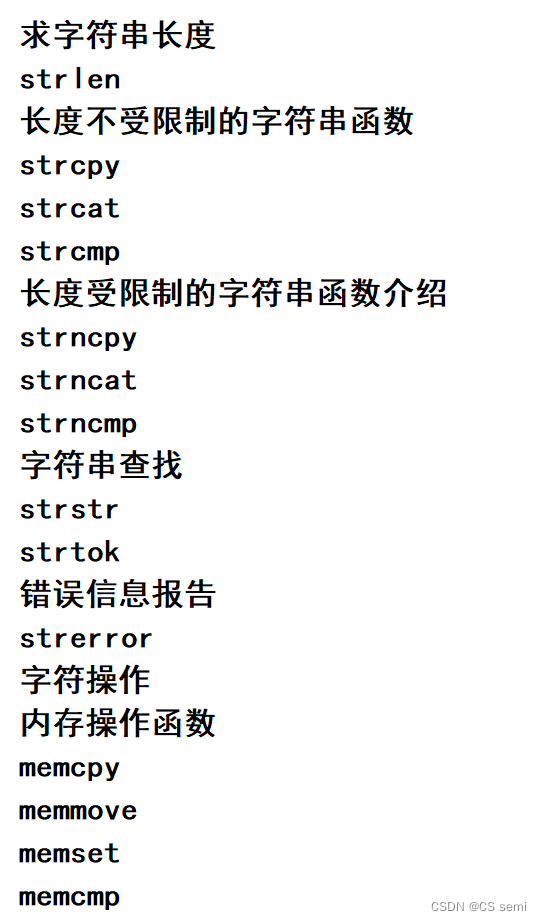C语言中对字符和字符串的处理很是频繁但是C语言本身是没有字符串类型的字符串通常放在常量字符串中或者字符数组中。字符串常量适用于那些对它不做修改的字符串函数.

# 一、strlen

## 一介绍

1.字符串已经 ‘\0’ 作为结束标志strlen函数返回的是在字符串中 ‘\0’ 前面出现的字符个数不包含 ‘\0’ )。
2.参数指向的字符串必须要以 ‘\0’ 结束。
3.注意函数的返回值为size_t是无符号的
4.学会strlen函数的模拟实现

``````#include<stdio.h>
int main() {
if (strlen("abc") - strlen("abcdef")) {
printf(">\n");
}
else {
printf("<=\n");
}
return 0;
}
``````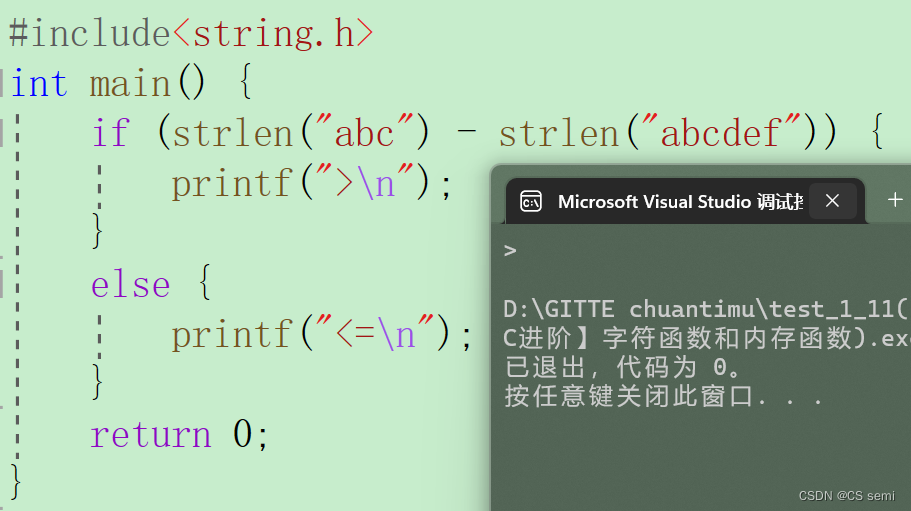## 二模拟实现

``````#include<assert.h>
#include<stdio.h>

//size_t == typedef unsigned int size_t
//法3
//指针-指针
size_t my_strlen(const char* str) {
assert(str);
const char* start = str;//定义首指针
while (*str) {
str++;
}
return str - start;//计算长度
}

//法2
//递归思想
size_t my_strlen(const char* str) {
assert(str);
if (*str != '\0')
return 1 + my_strlen(str + 1);
else
return 0;
}

//法1
//常规思路往后加1找大小
size_t my_strlen(const char* str) {
assert(str);
int count = 0;
while (*str) {
str++;
count++;
}
return count;
}

int main() {
char arr[] = "abcdef";
int len = my_strlen(arr);
printf("%d\n", len);
return 0;
}
``````

# 二、strcpy

<<点此>>传送门

## 一介绍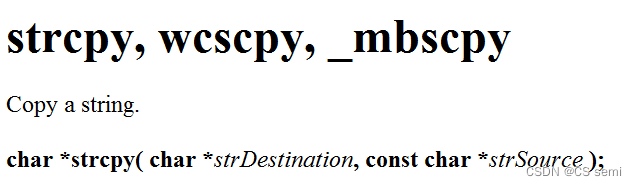1.源字符串必须以 ‘\0’ 结束。 ---- 将strSource中一直到’\0’之前的字符以及’\0’拷贝到strDestination所以一定要有’\0’。
2.会将源字符串中的 ‘\0’ 拷贝到目标空间。 — 从strSource中的所有字符包括’\0’都拷贝上去。
3.目标空间必须足够大以确保能存放源字符串。 — 被拷贝的空间一定要是足够大的空间能够存放拷贝的字符串。
4.目标空间必须可变。— 如果给的是一个常量字符串那肯定用不了strcpy了只有数组是可变的才能用strcpy。

## 二模拟实现

``````#include<stdio.h>
#include<assert.h>
char* my_strcpy(char* dest, const char* src) {
assert(dest && src);
char* ret = dest;
while (*dest++ = *src++) {
;
}
return dest;
}

int main() {
char arr1 = "*************";
char arr2[] = "abcdef";
my_strcpy(arr1, arr2);
printf("%s\n", arr1);
return 0;
}
``````

# 三、strcat

## 一介绍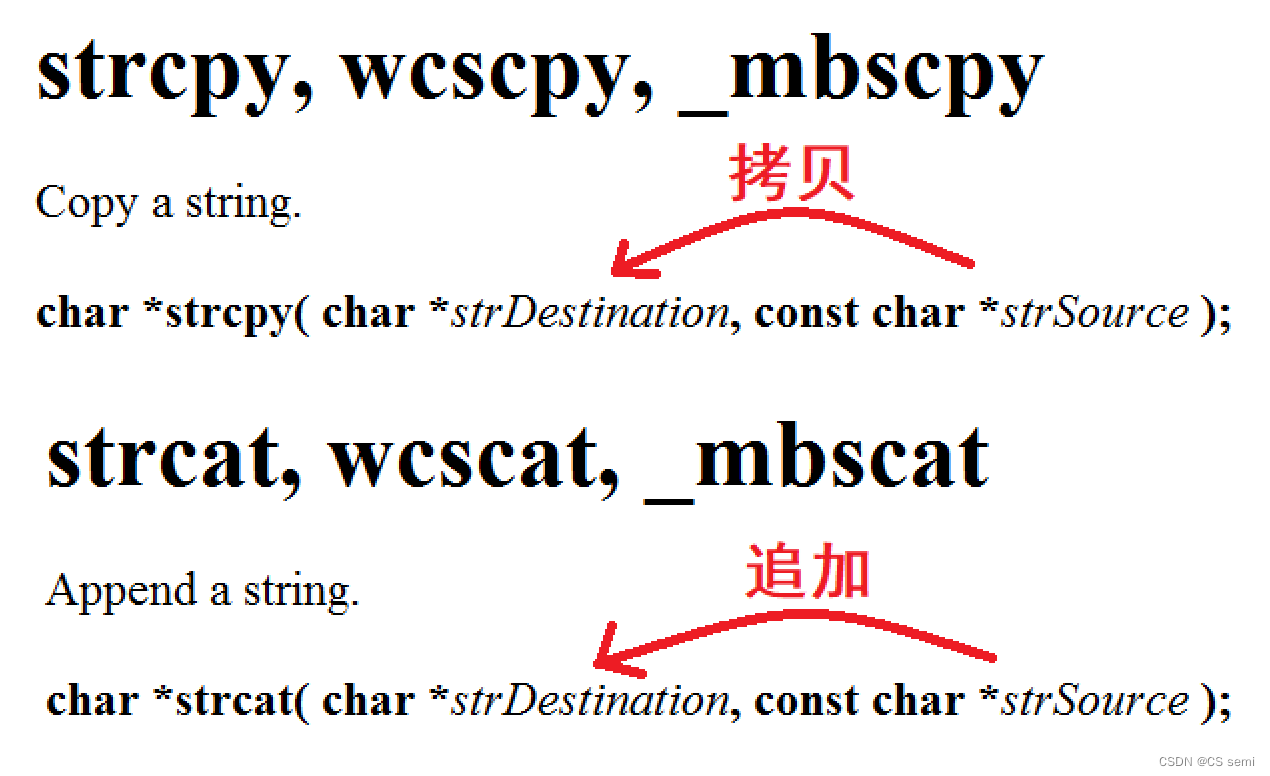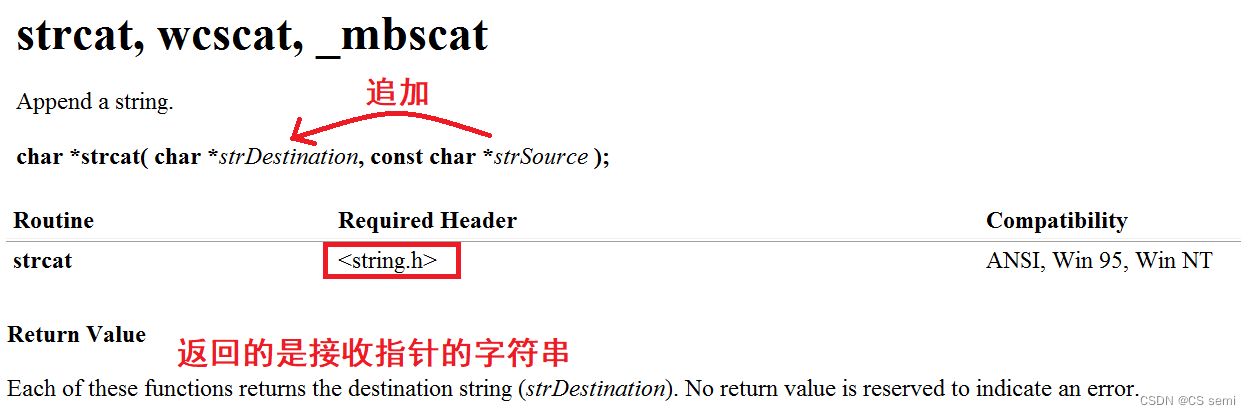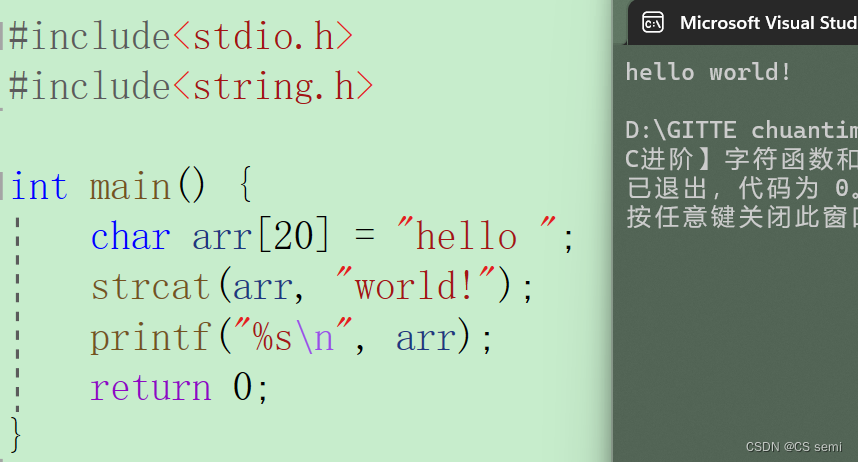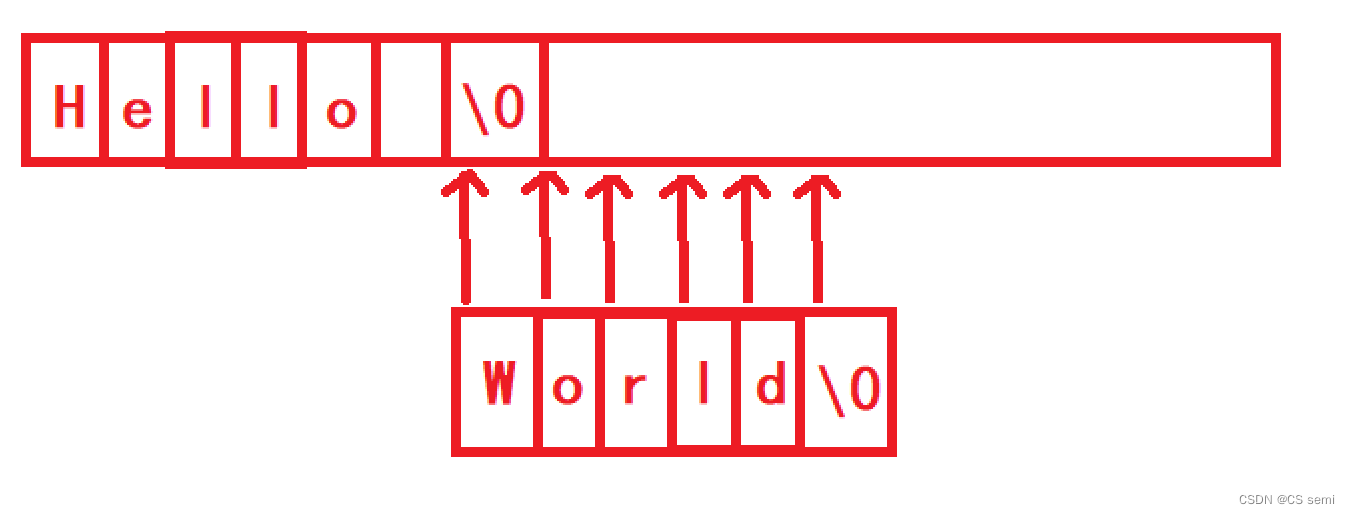1.源字符串必须以 ‘\0’ 结束。
2.目标空间必须有足够的大能容纳下源字符串的内容。
3.目标空间必须可修改。

## 二模拟实现

``````#include<stdio.h>
#include<assert.h>
#include<string.h>
char* my_strcat(char* dest, char* src) {
assert(dest && src);
char* ret = dest;
//1.找到目标空间的'\0'
while (*dest != '\0') {
dest++;
}
//2.追加
while (*dest++ = *src++) {
;
}
return ret;
}

int main() {
char arr = "hello ";
char arr1[] = "world!";
//char* p = "world!";
my_strcat(arr, arr1);
printf("%s\n", arr);
return 0;
}
``````

## 三有趣的知识

strcat库函数能够进行追加字符串那它自己追加自己呢答案是死循环如下图解释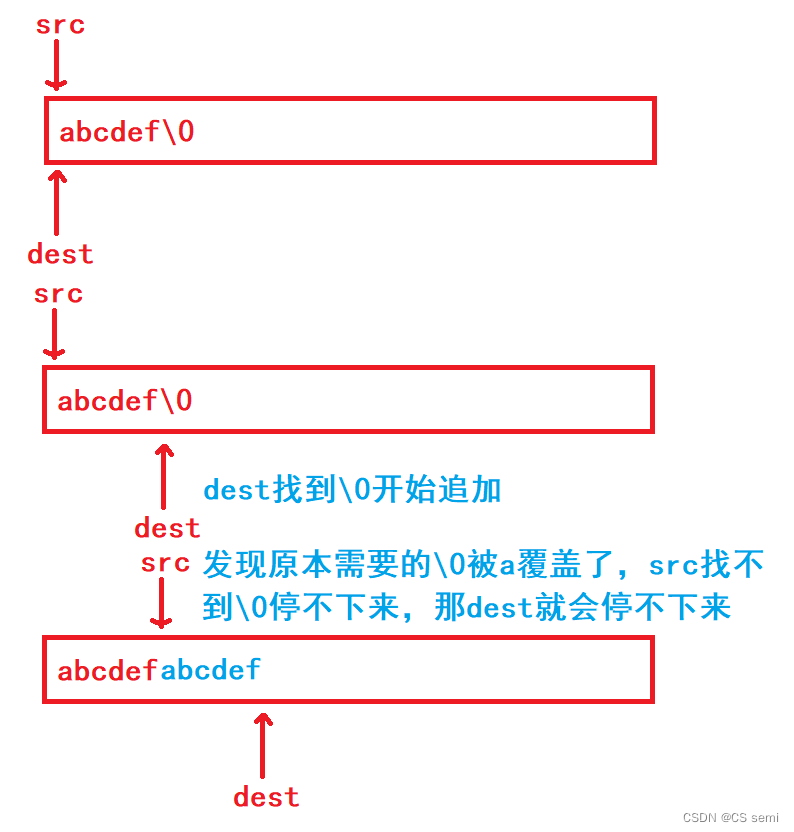# 四、strcmp

## 一介绍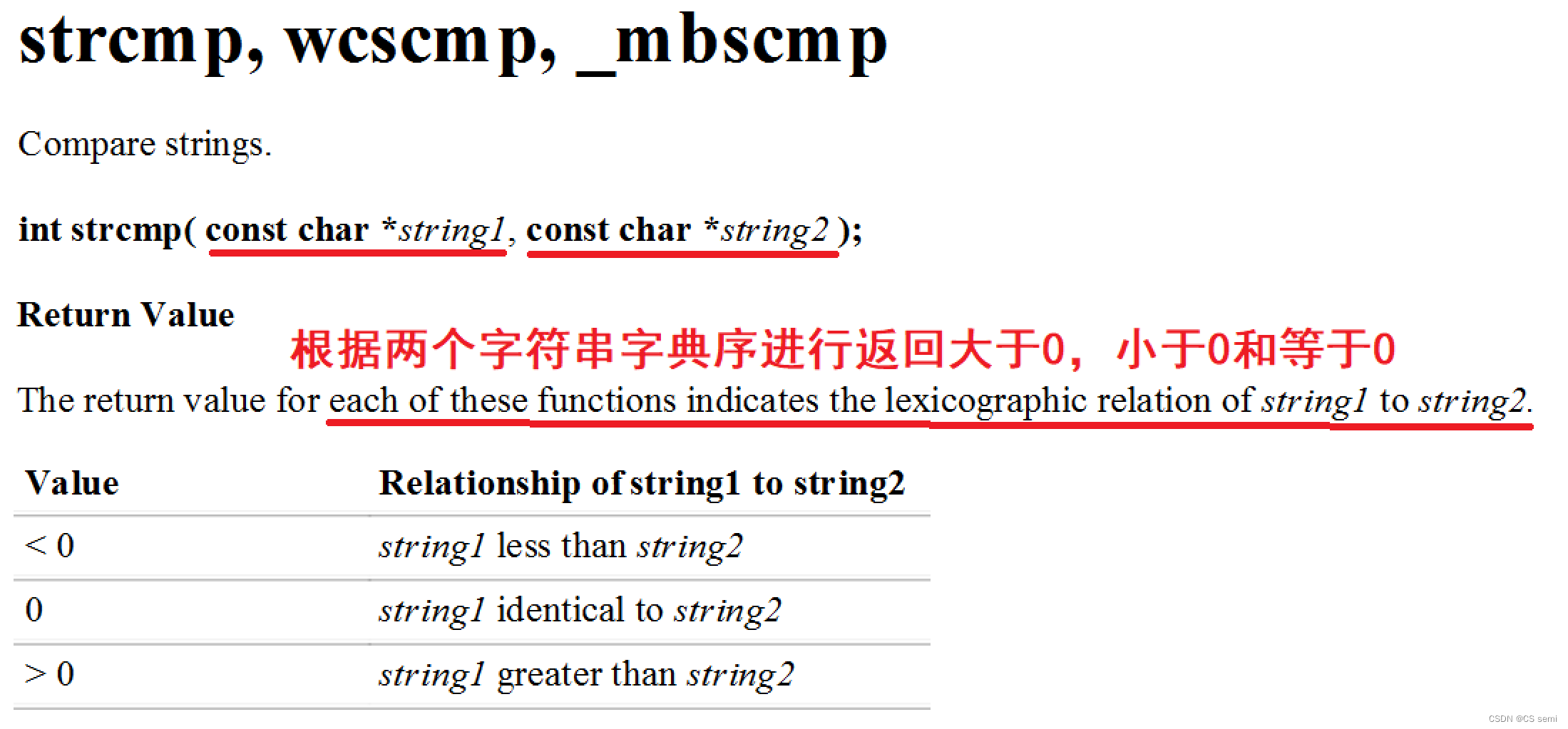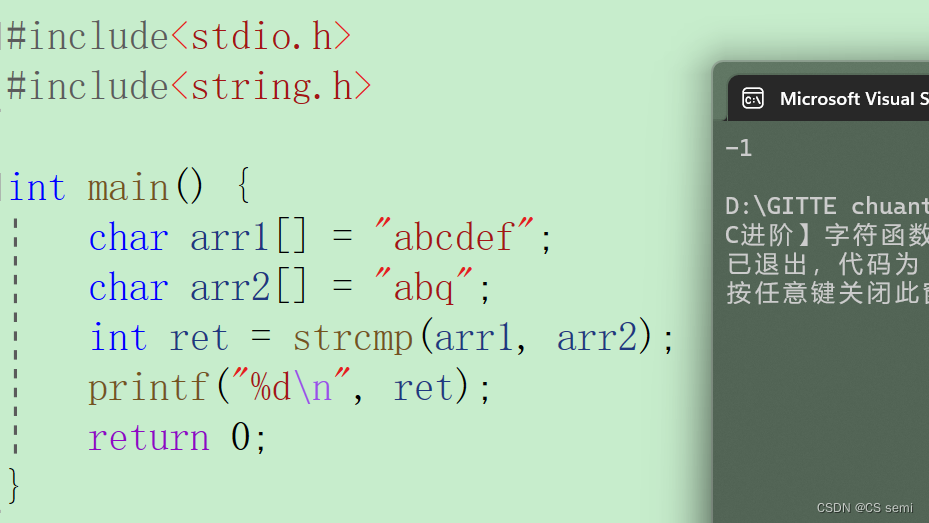ps:在VS环境下>返回1<返回-1=返回0。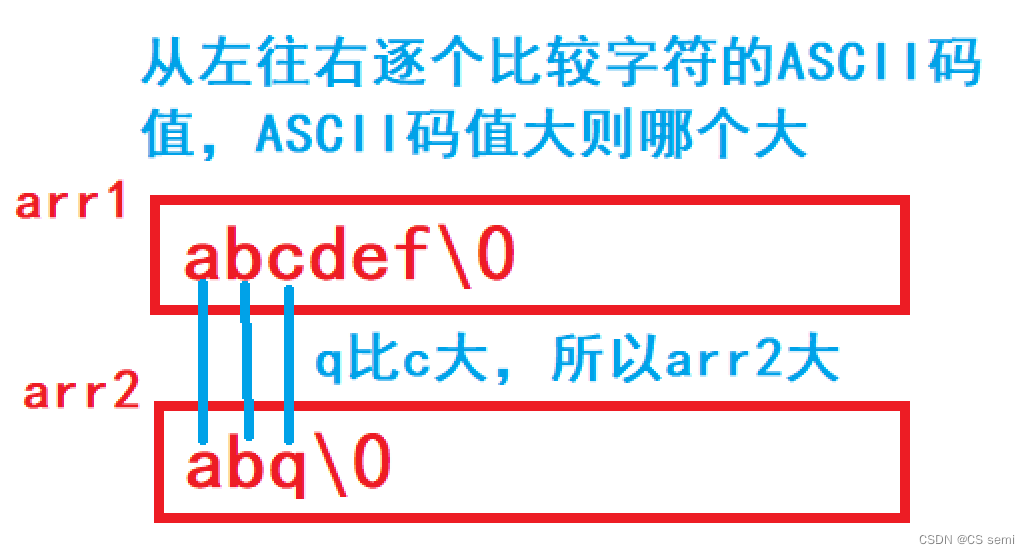## 二模拟实现

``````//VS环境下
#include<stdio.h>
#include<assert.h>
int my_strcmp(const char* str1, const char* str2) {
assert(str1 && str2);
while (*str1 == *str2) {
if (*str1 == '\0' && *str2 == '\0') {//两个字符串相等的时候
return 0;
}
str1++;
str2++;
}
if (*str1 > *str2)
return 1;
else
return -1;
}
int main() {
char arr1[] = "abcdef";
char arr2[] = "abq";
int ret = my_strcmp(arr1, arr2);
printf("%d\n", ret);
return 0;
}
``````

``````#include<stdio.h>
#include<assert.h>
int my_strcmp(const char* str1, const char* str2) {
assert(str1 && str2);
while (*str1 == *str2) {
if (*str1 == '\0' && *str2 == '\0') {//两个字符串相等的时候
return 0;
}
str1++;
str2++;
}
/*if (*str1 > *str2)
return 1;
else
return -1;*/
return *str1 - *str2;
}
int main() {
char arr1[] = "abcdef";
char arr2[] = "abq";
int ret = my_strcmp(arr1, arr2);
printf("%d\n", ret);
return 0;
}
``````

# 五、strncpy

## 一介绍

1.拷贝num个字符从源字符串到目标空间。
2.如果源字符串的长度小于num则拷贝完源字符串之后在目标的后边追加0直到num个。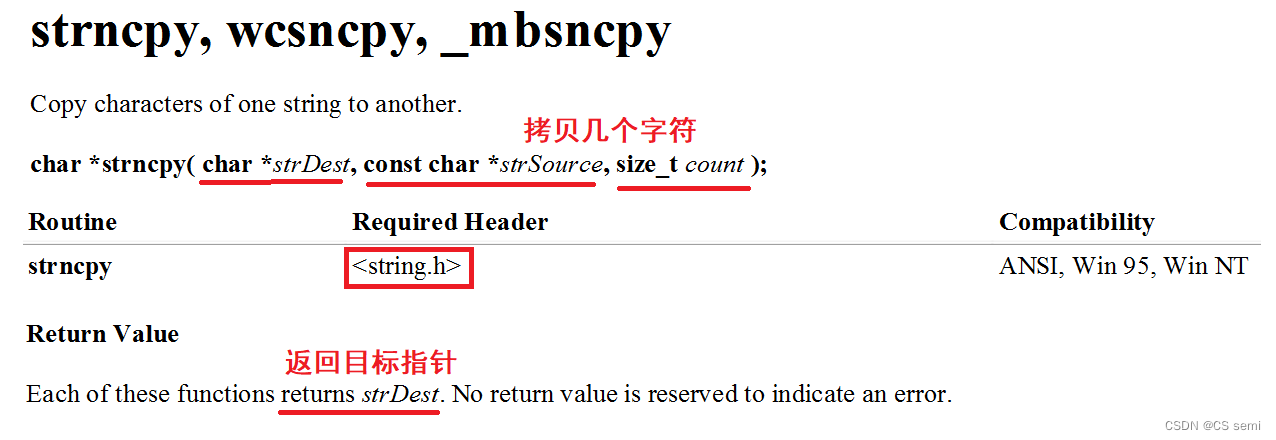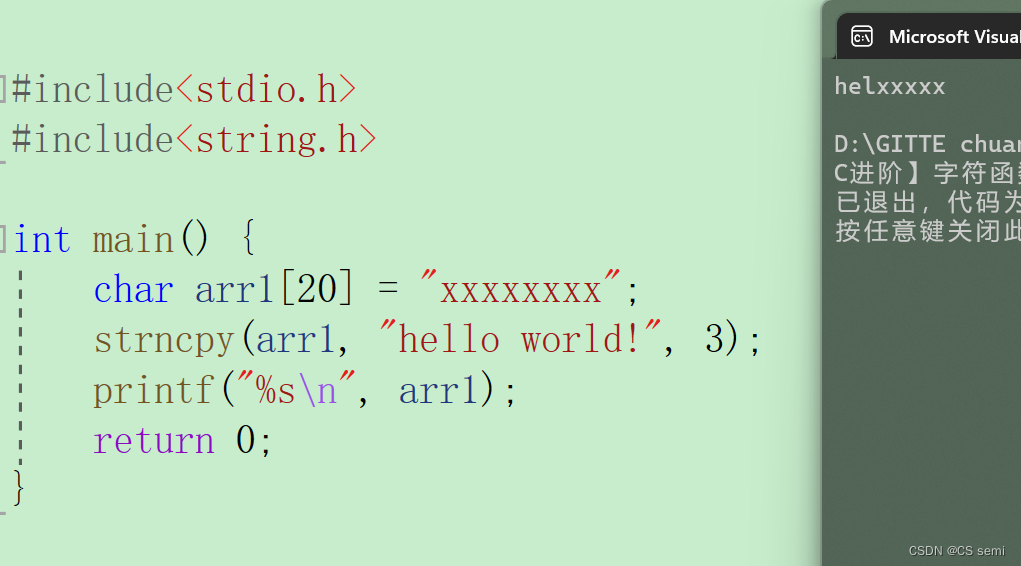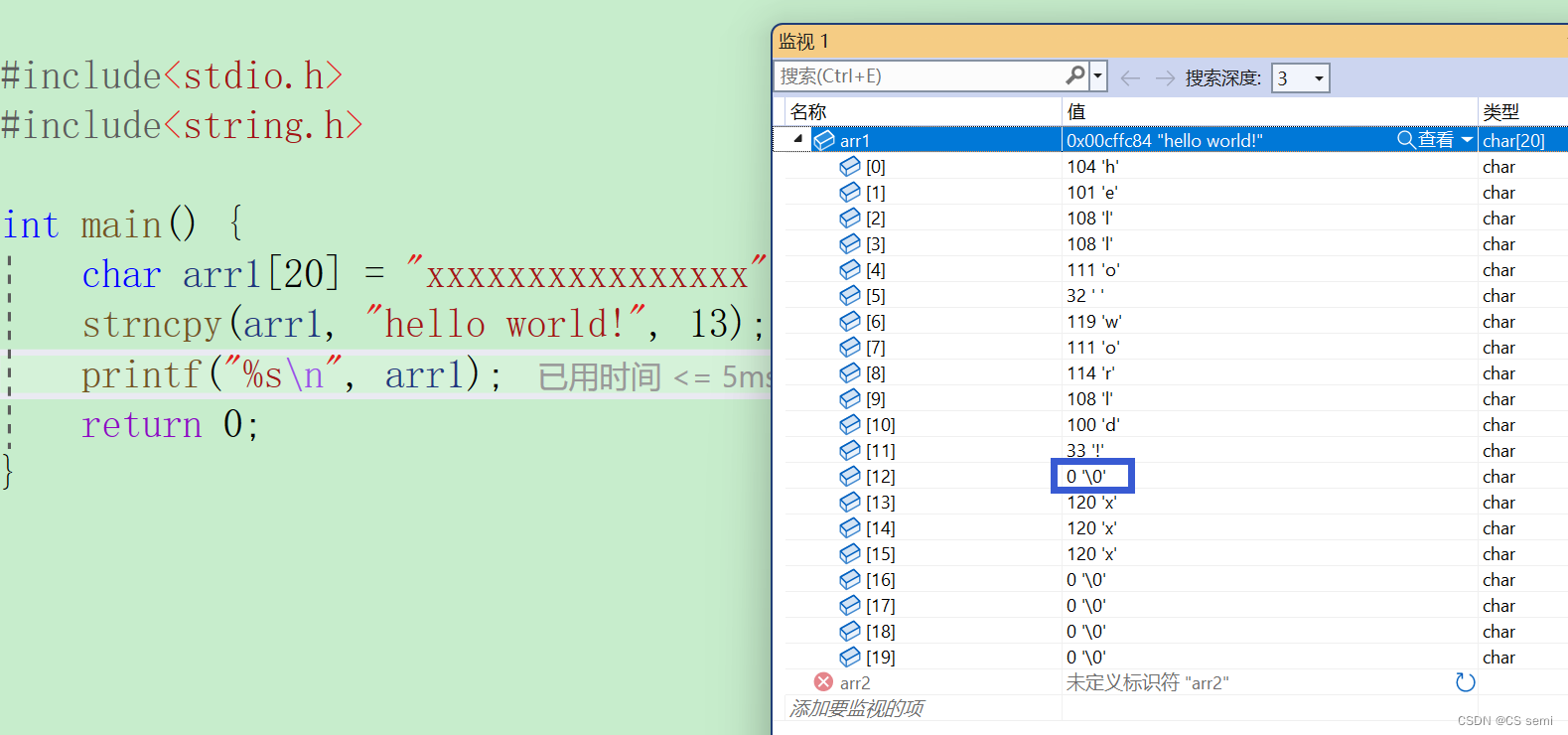## 二模拟实现

``````#include<stdio.h>
#include<assert.h>
char* my_strncpy(char* dest, const char* src, size_t num) {
assert(dest && src);
char* ret = dest;
while (num--) {
*dest++ = *src++;
}
return ret;
}
int main() {
char arr1 = "xxxxxxxx";
char* ret = my_strncpy(arr1, "hello world!", 3);
printf("%s\n", arr1);
return 0;
}
``````

# 六、strncat

## 一介绍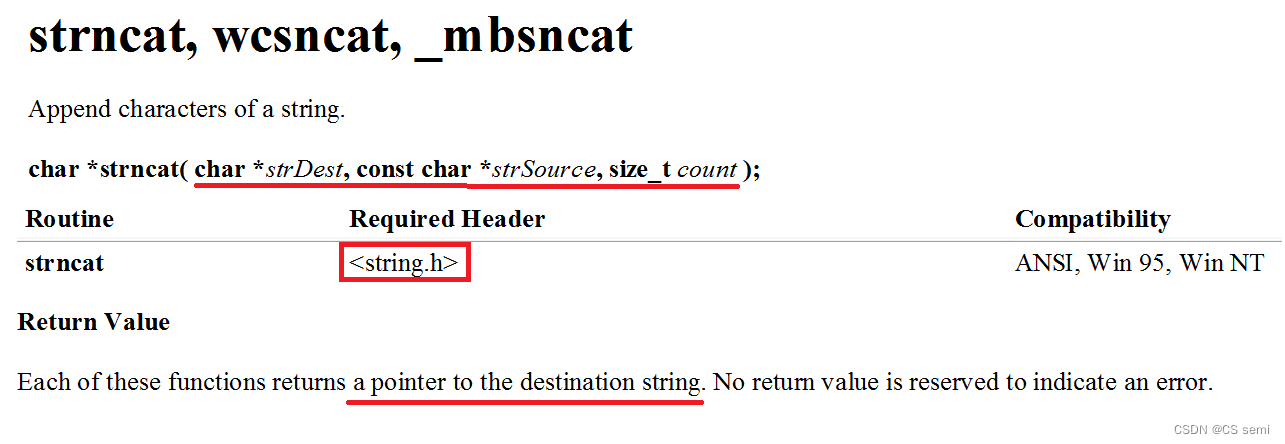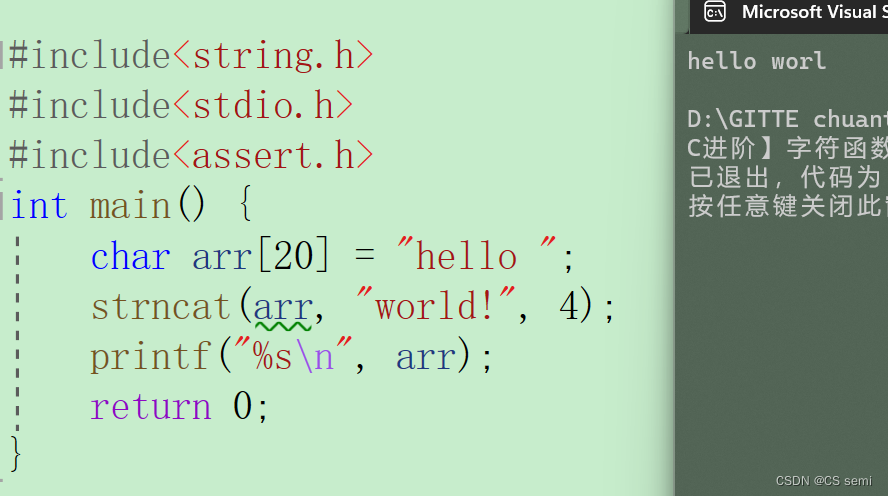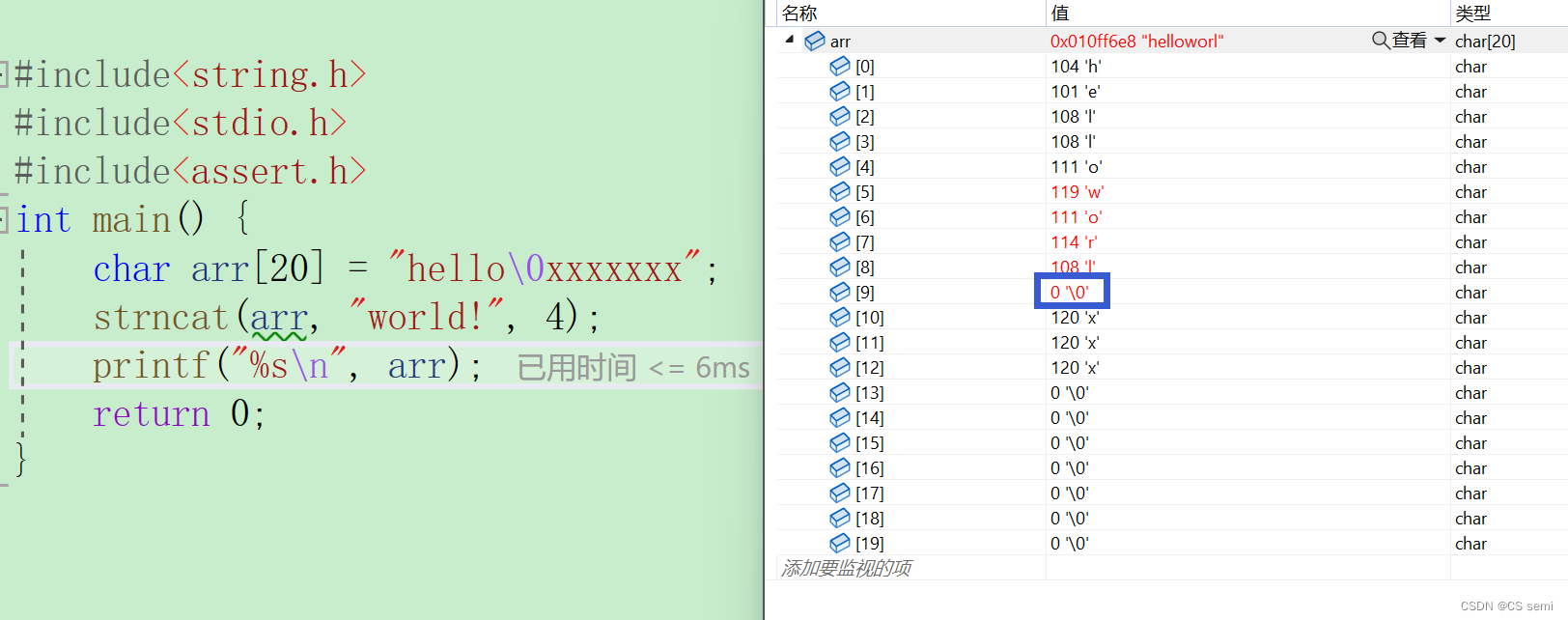## 二模拟实现

``````#include<stdio.h>
#include<assert.h>
char* my_strncat(char* dest, char* src, size_t num) {
assert(dest && src);
char* ret = dest;
//1.找到目标空间的'\0'
while (*dest != '\0') {
dest++;
}
//2.追加
while (num--) {
*dest++ = *src++;
}
*dest = 0;//\0后面的值都不要'\0'的ASCII码值为0
return ret;
}
int main() {
char arr = "hello\0xxxxxxx";
char* ret = my_strncat(arr, "world!", 4);
printf("%s\n", arr);
return 0;
}
``````

# 七、strncmp

## 一介绍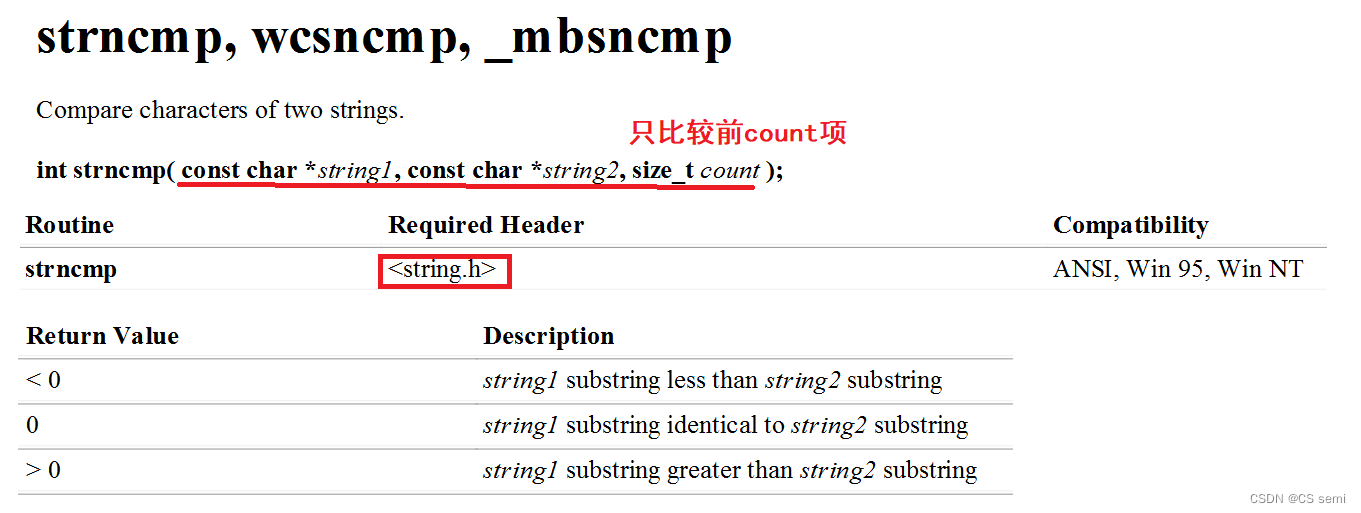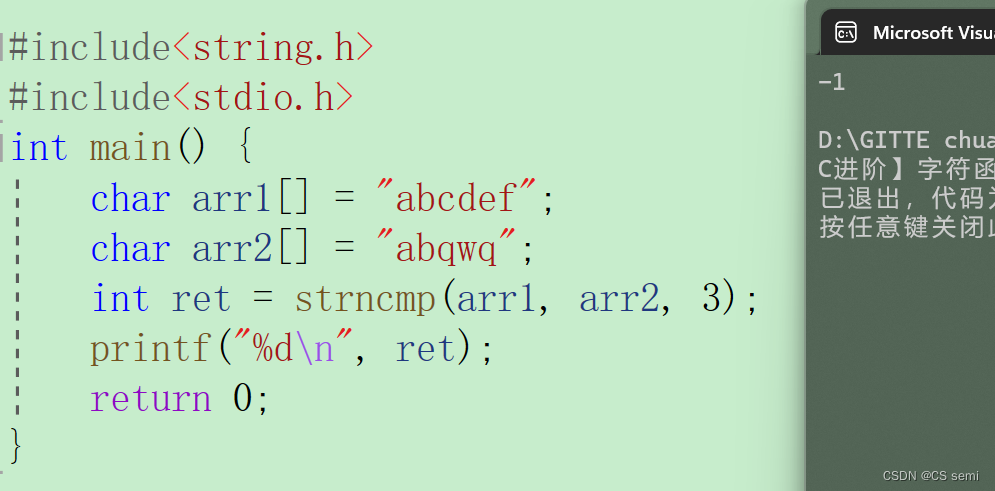## 二模拟实现

``````#include<stdio.h>
#include<assert.h>
int my_strncmp(char* str1, char* str2, int num) {
assert(str1 && str2);
while (num--) {
if (*str1 == *str2) {
str1++;
str2++;
}
else {
if (*str1 > *str2) {
return 1;
}
else {
return -1;
}
}
}
return 0;
}
int main() {
char arr1[] = "abcdef";
char arr2[] = "abqwq";
int ret = my_strncmp(arr1, arr2, 3);
printf("%d\n", ret);
return 0;
}
``````

# 八、strstr

## 一介绍

strstr库函数简单来讲就是找在第一个字符串找有没有第二个字符串的地址有则返回第一个出现的字符串的位置无则返回空指针。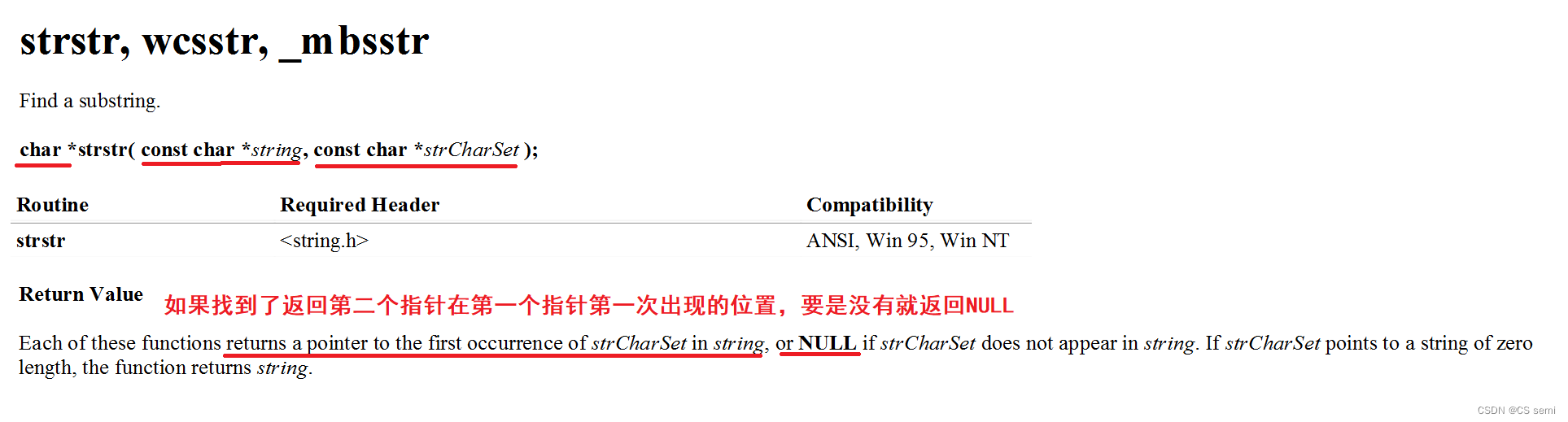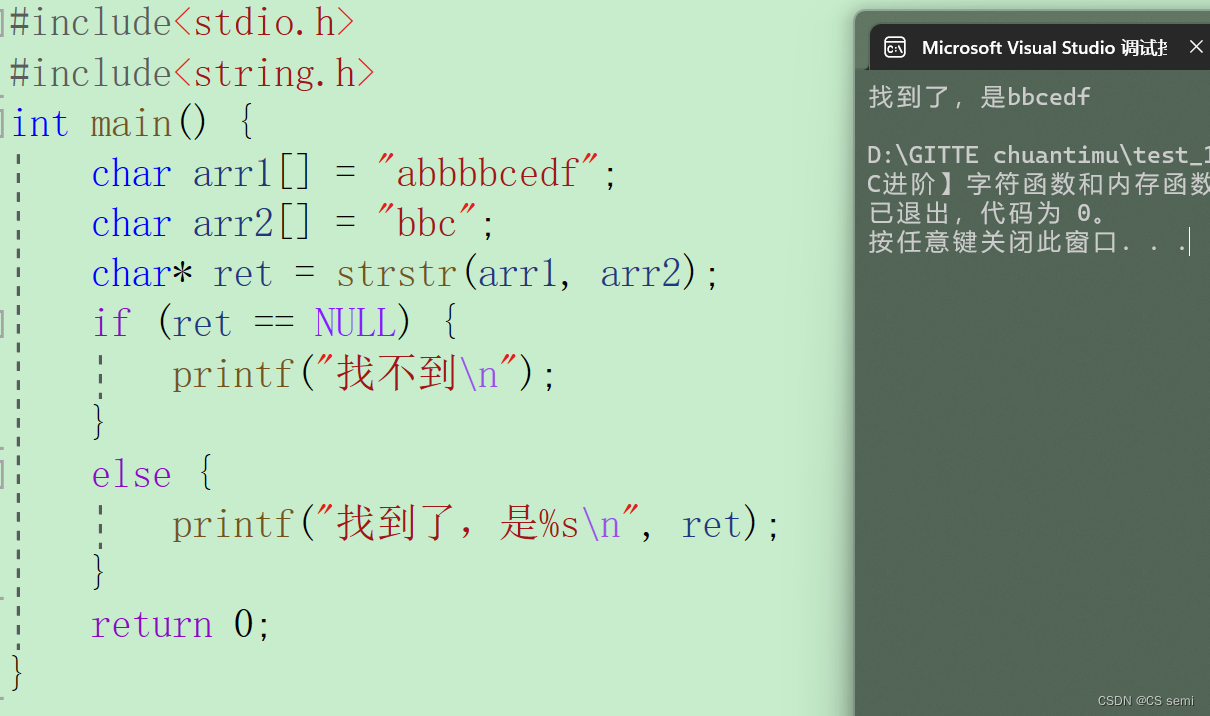## 二模拟实现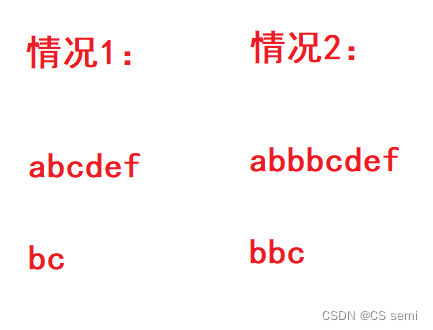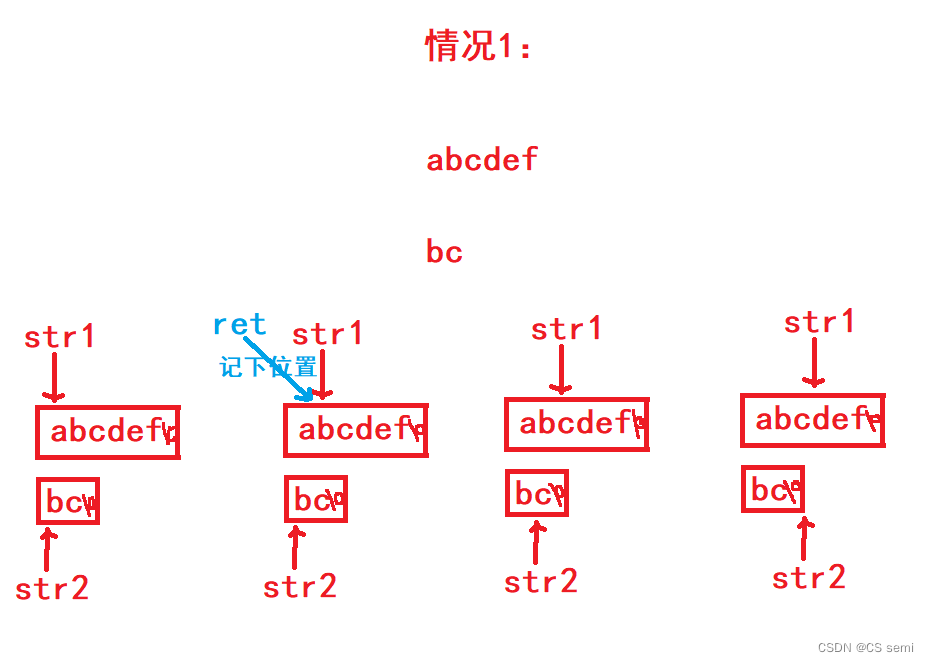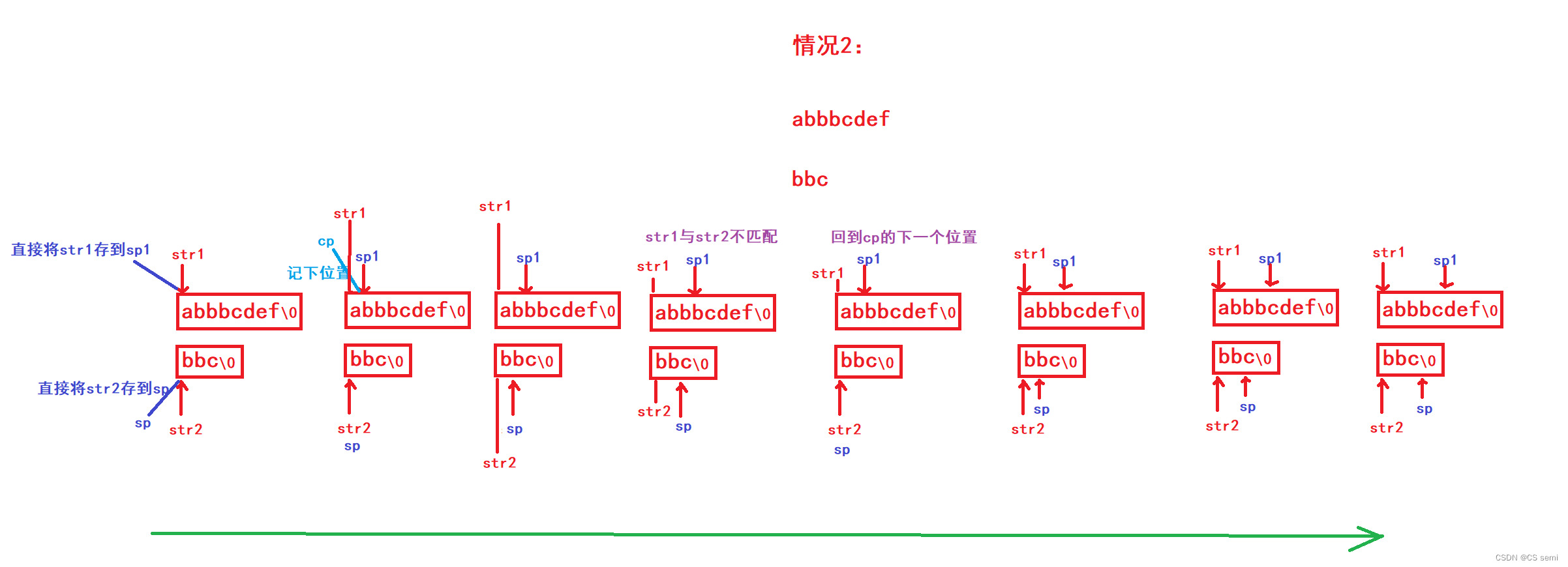``````#include<stdio.h>
#include<assert.h>
char* my_strstr(const char* str1, const char* str2) {
assert(str1 && str2);
//空字符串找不到但输出是第一个字符串
if (*str2 == '\0') {
return str1;
}
//找替代的指针让它们去走
const char* sp1 = str1;
const char* sp = str2;
//cp去储存每次str1与str2两个一样的地址
const char* cp = str1;

while (*cp) {
//碰到一样元素后续不一样sp1先回来再往后走一位
//找不到sp永远是处在str2首地址的位置
sp1 = cp;
sp = str2;
while (*sp1!='\0' && *sp!='\0' && *sp1 == *sp) {//判断都不是走到末尾
sp1++;
sp++;
}
if (*sp == '\0') {//第二个字符串走到头
return cp;
}
cp++;
}
return NULL;

}
int main() {
char arr1[] = "abbbbcedf";
char arr2[] = "bbc";
char* ret = my_strstr(arr1, arr2);
if (ret == NULL) {
printf("找不到\n");
}
else {
printf("找到了是%s\n", ret);
}
return 0;
}
``````

# 九、strtok

## 一介绍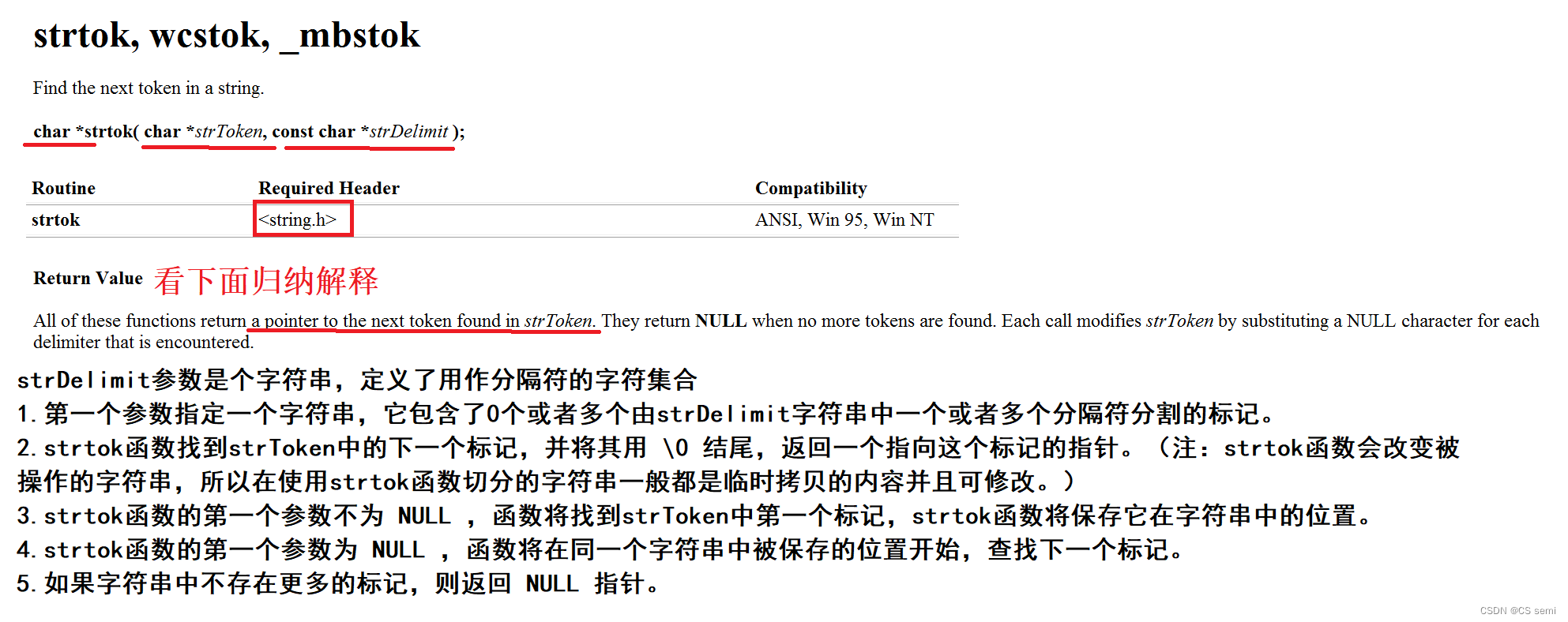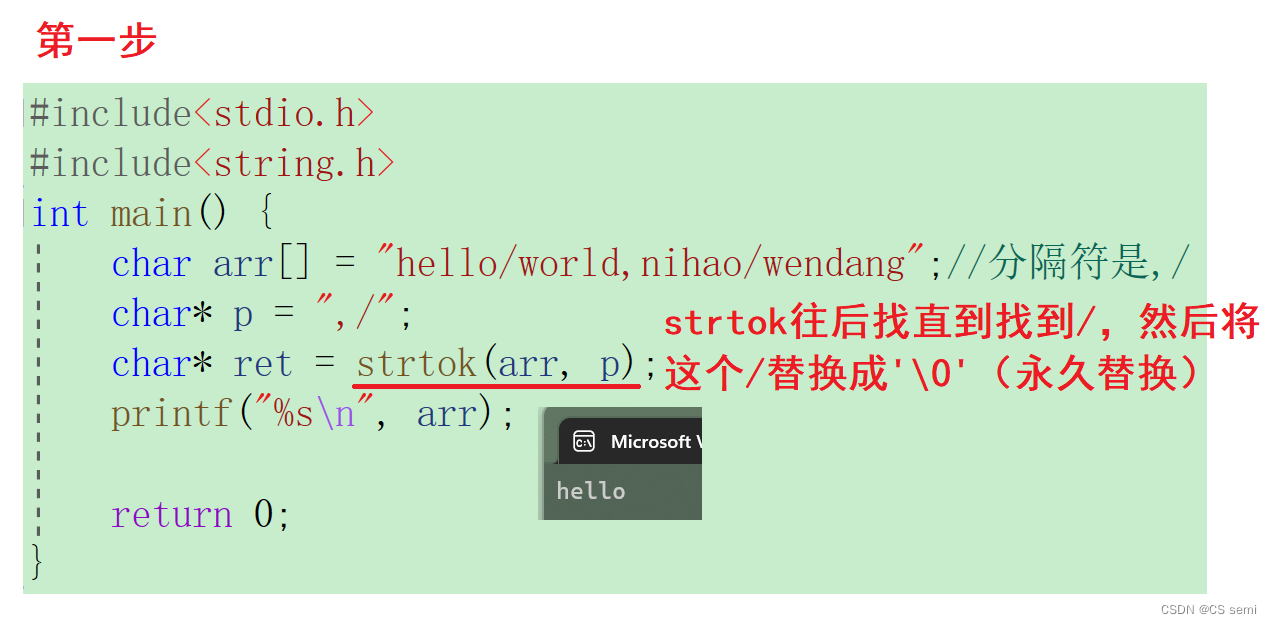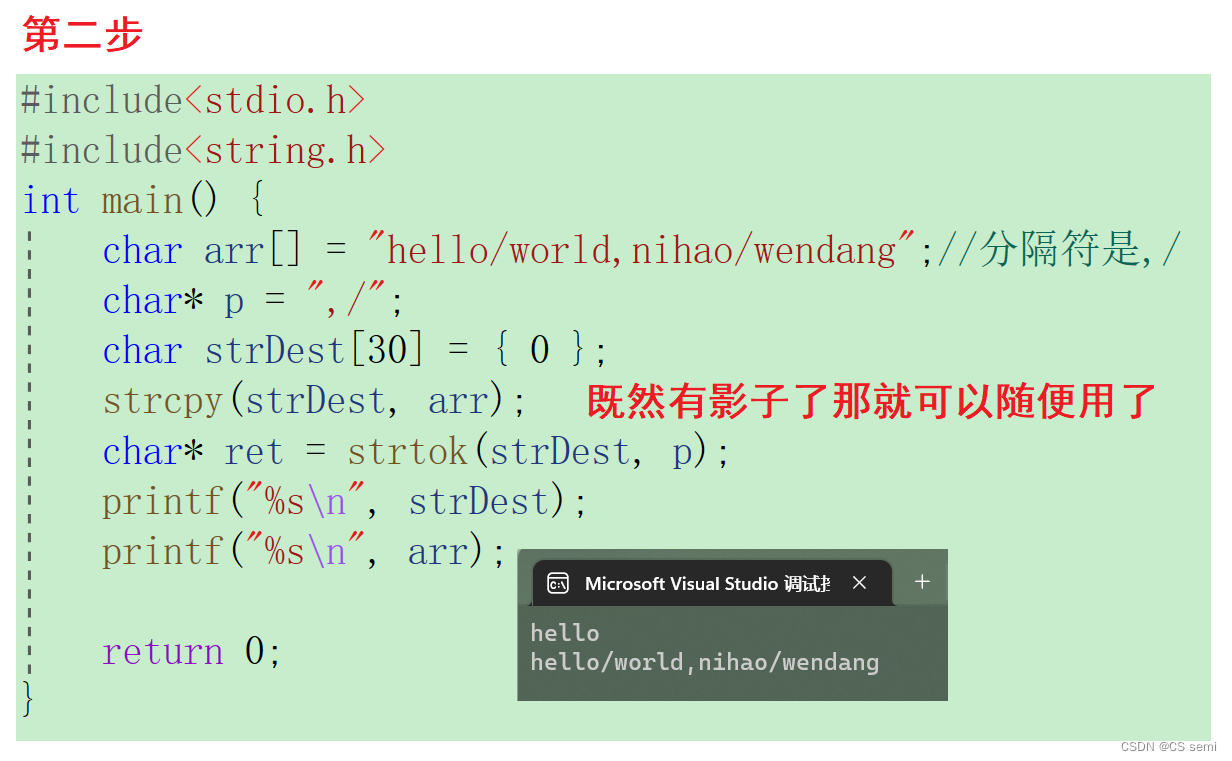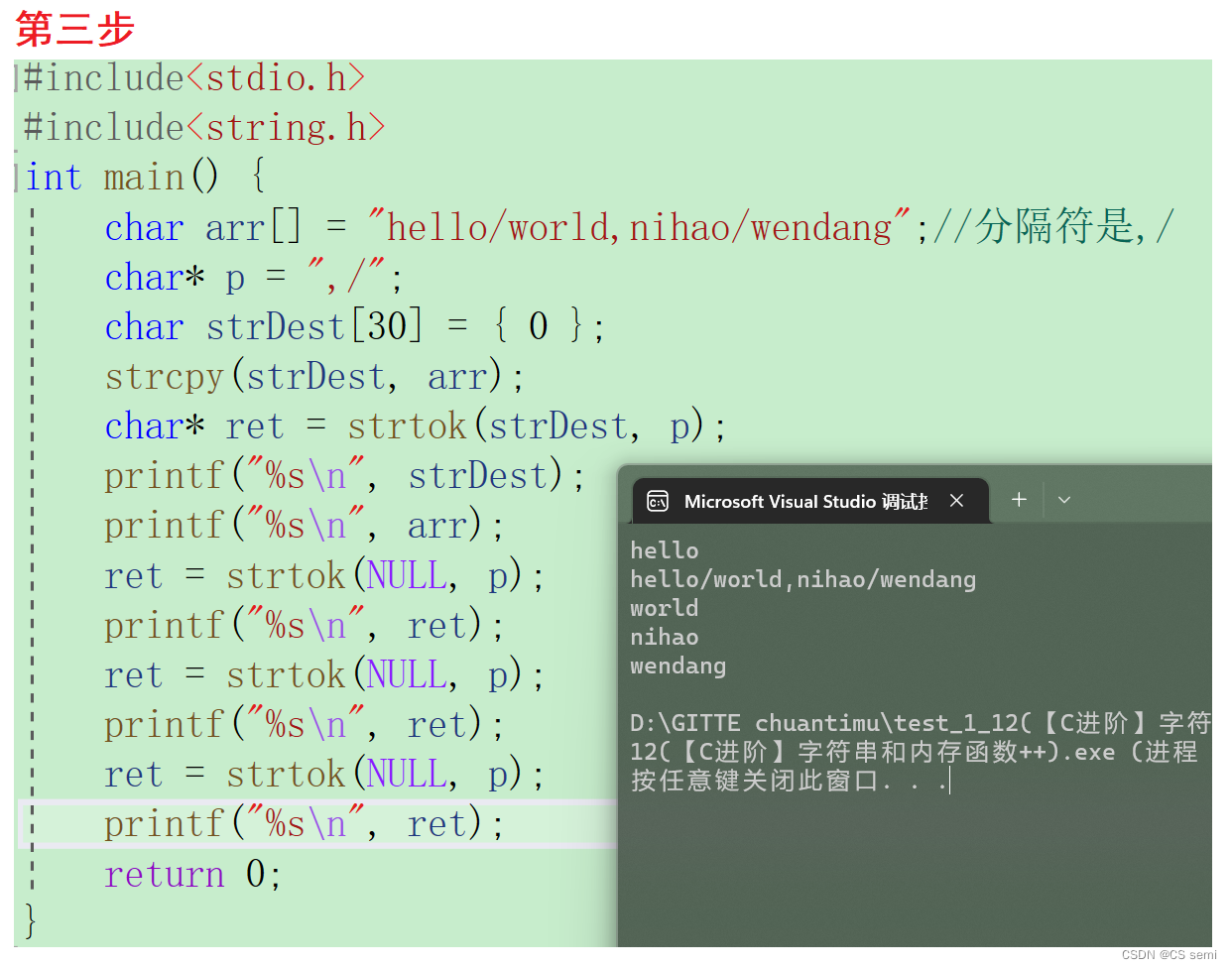``````#include<stdio.h>
#include<string.h>
int main() {
char arr[] = "hello/world,nihao/wendang";//分隔符是,/
char* p = ",/";
char strDest = { 0 };
strcpy(strDest, arr);
char* ret = strtok(strDest, p);
printf("%s\n", strDest);
printf("%s\n", arr);
ret = strtok(NULL, p);
printf("%s\n", ret);
ret = strtok(NULL, p);
printf("%s\n", ret);
ret = strtok(NULL, p);
printf("%s\n", ret);
return 0;
}
``````

``````#include<stdio.h>
#include<string.h>
int main() {
char arr[] = "hello/world,nihao/wendang";//分隔符是,/
char* p = ",/";
char strDest = { 0 };
strcpy(strDest, arr);
char* ret = NULL;
for(ret = strtok(strDest, p); ret != NULL; ret = strtok(NULL, p)) {
printf("%s\n", ret);
}

return 0;
}
``````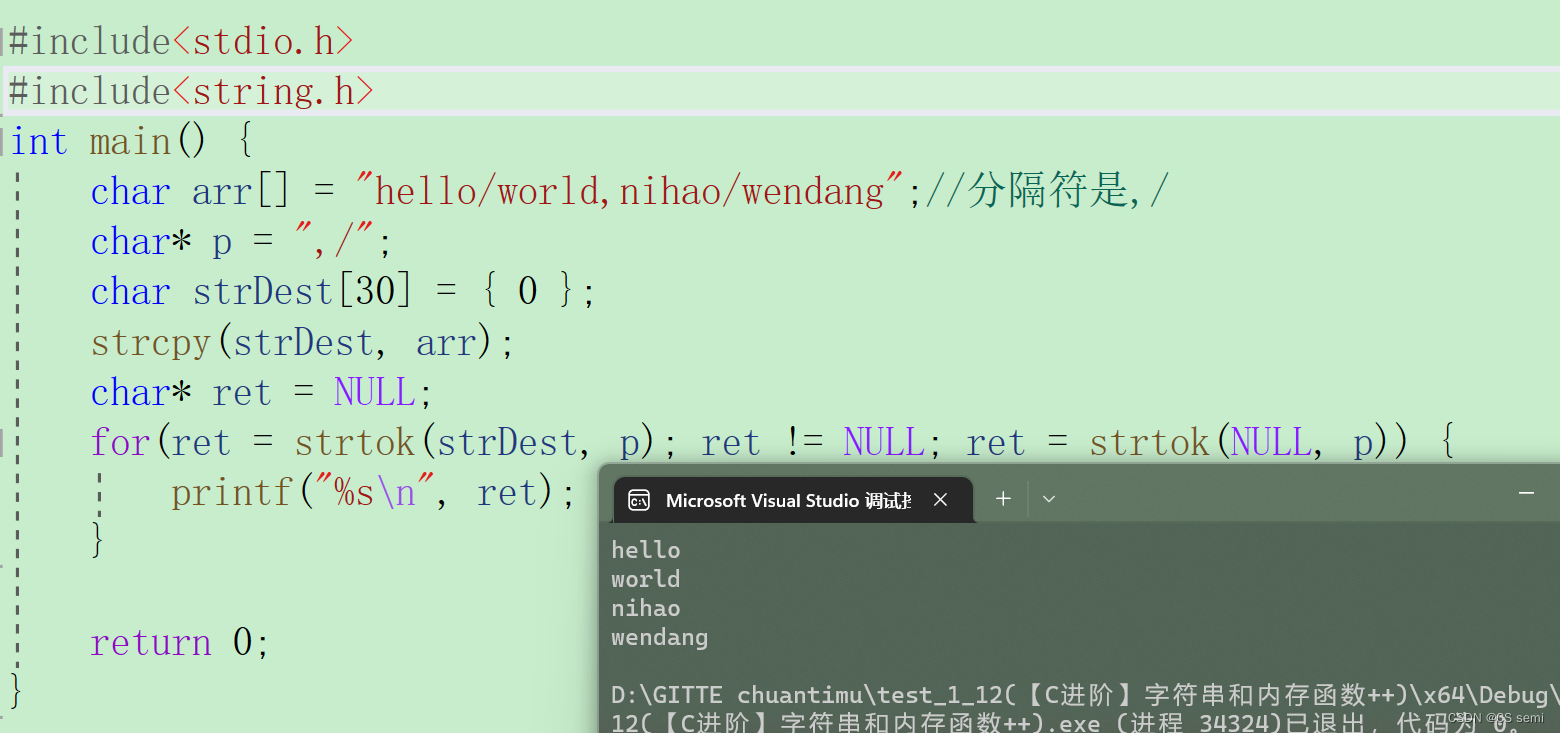## 二模拟实现

``````#include<stdio.h>
#include<string.h>
#include<assert.h>

char* my_strtok(char* strToken, const char* strDelimit){
assert(strDelimit && strDelimit);
static char* s1 = NULL;//静态变量之后再进来的时候可以接着原本的值使用
static char* s2 = NULL;
static int len1 = 0;
static int count = 0;
int len2 = 0;
int i = 0;
if (strToken) { //第一个字符不等于'\0'
s1 = strToken;
len1 = strlen(strToken);
len2 = strlen(strDelimit);
while(*strToken != '\0'){//解引用以后拿到的字符不等于'\0'
for (i = 0; i < len2; i++) {
if (i == 0){
count++;
}
if (*strToken == *(strDelimit + i)){
*strToken = '\0'; //找到了那个分隔符就将这个分隔符内容赋为0
s2 = strToken;
return s1;//返回头指针
}
}
strToken++;
}
}
else{
s1 = s2 + 1; //s1去移动s2不用运动
len2 = strlen(strDelimit);
strToken = s1;
while(*strToken != '\0'){
for (i = 0; i < len2; i++){
if (i == 0){
count++;
}
if (*strToken == *(strDelimit + i)){
*strToken = '\0';   //找到了那个分隔符就将这个分隔符内容赋为0
s2 = strToken;
return s1;
}
}
strToken++;
}
}
if (count > len1){//要是
return NULL;
}
return s1;
}

int main(){
char arr1[] = "hello/world,nihao/wendang";
char arr2[] = "/,";
char* str = NULL;
for (str = my_strtok(arr1, arr2); str != NULL; str = my_strtok(NULL, arr2)){//进入循环不断的返回
printf("%s\n", str);
}
return 0;
}
``````

# 十、strerror

## 一介绍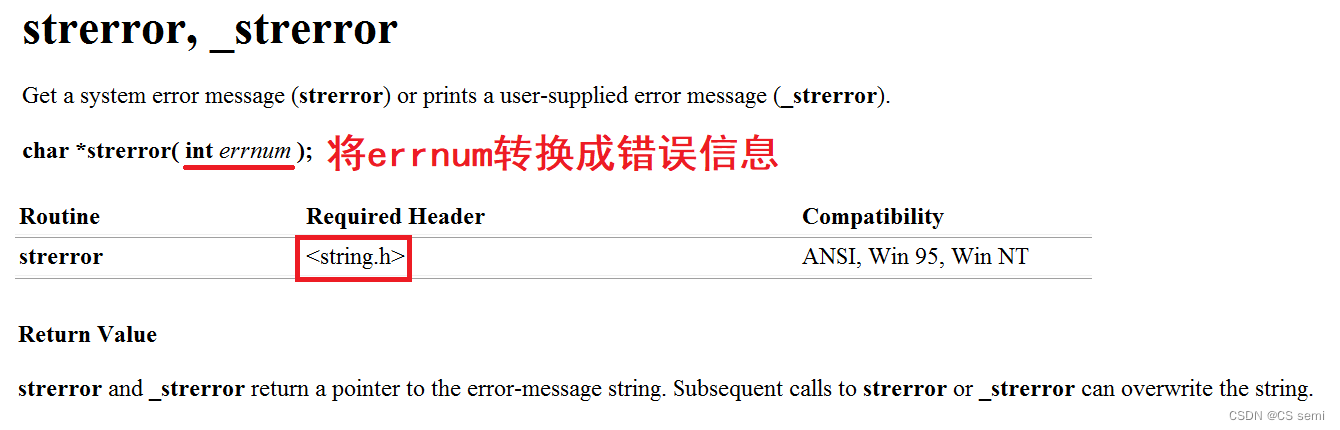C语言的库函数在运行的时候如果发生错误就会将错误码存放在一个变量中这个变量是errno错误码是一些数字所以我们需要将错误码翻译成错误信息返回错误码所对应的错误信息。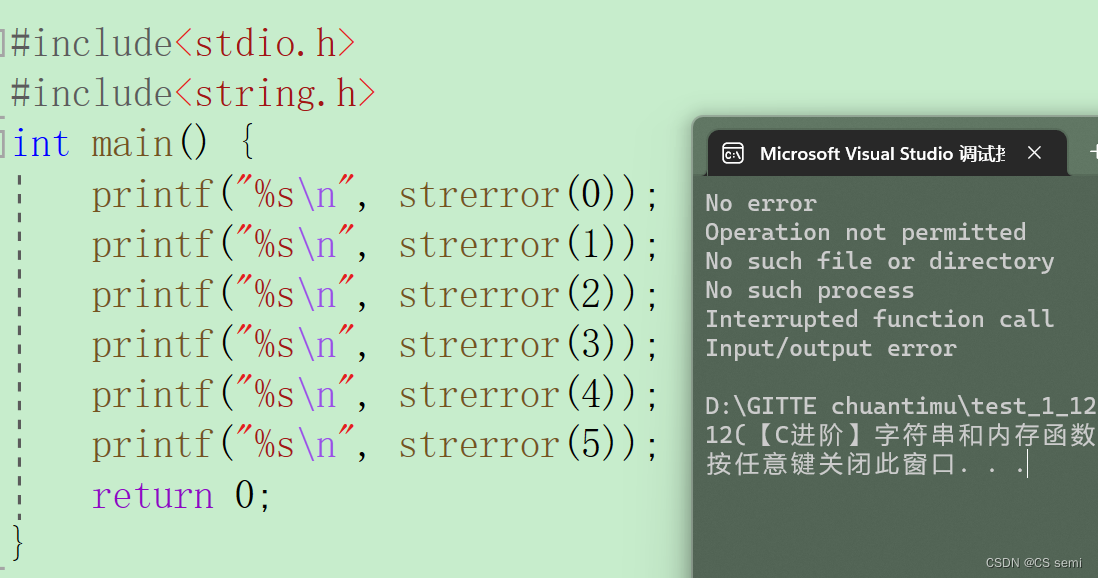## 二使用

``````#include<stdio.h>
#include<errno.h>
int main() {
//打开文件
FILE* pf = fopen("test.txt", "r");
if (pf == NULL) {
printf("%s\n",strerror(errno));//errno是用来保存错误信息strerror是用来进行译码
return 1;
}
//读文件
//关闭文件
fclose(pf);//释放
pf = NULL;

return 0;
}
``````

## 三perror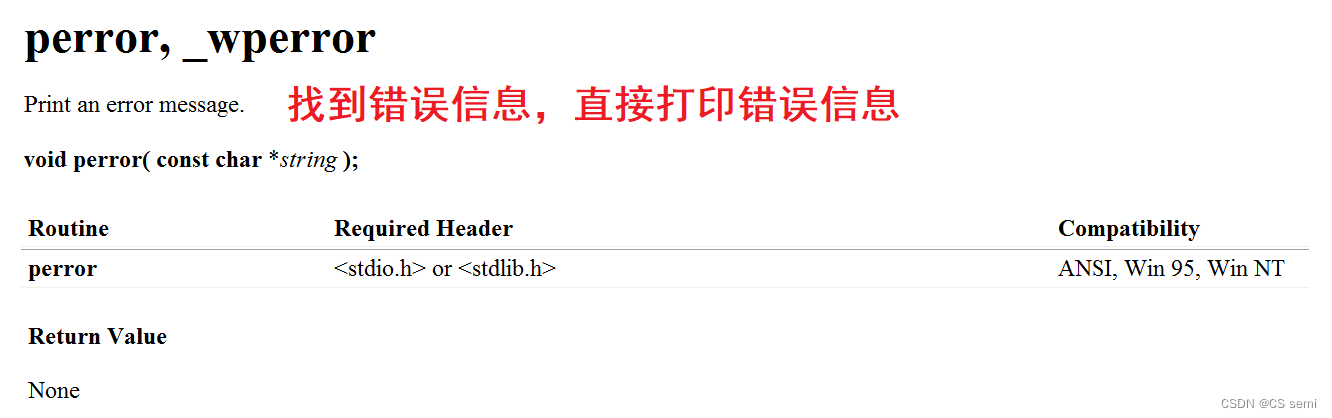``````#include<stdio.h>
int main() {
//打开文件
FILE* pf = fopen("test.txt", "r");
if (pf == NULL) {
//printf("%s\n",strerror(errno));
perror("fopen");
return 1;
}
//读文件
//关闭文件
fclose(pf);//释放
pf = NULL;

return 0;
}
``````

# 十一字符分类函数头文件<ctype.h>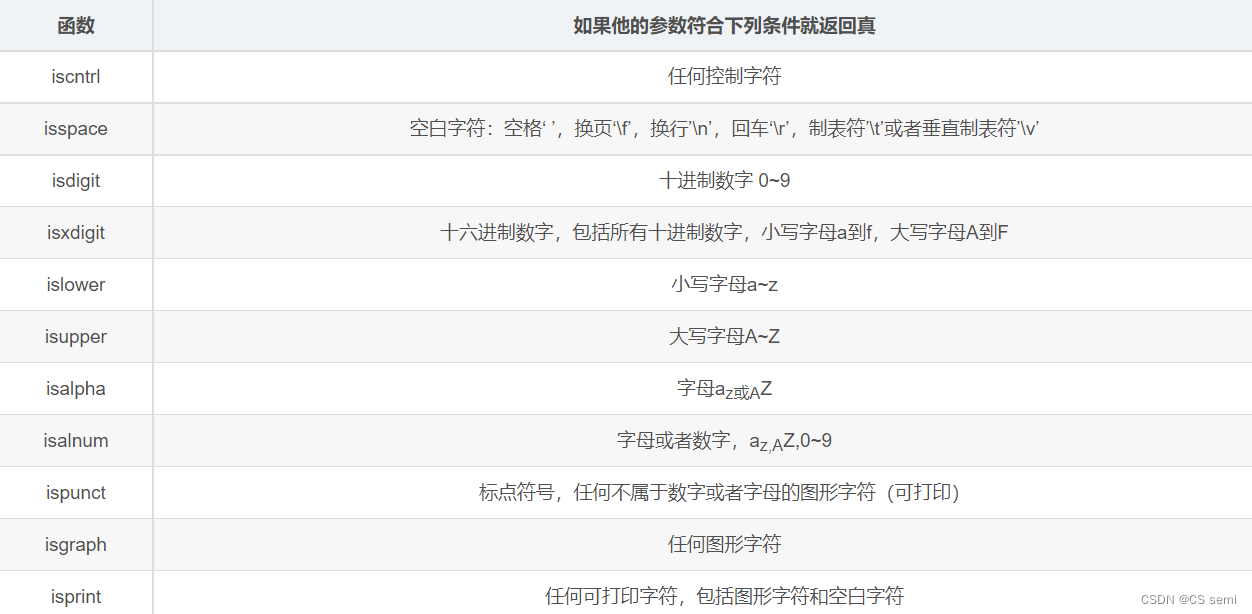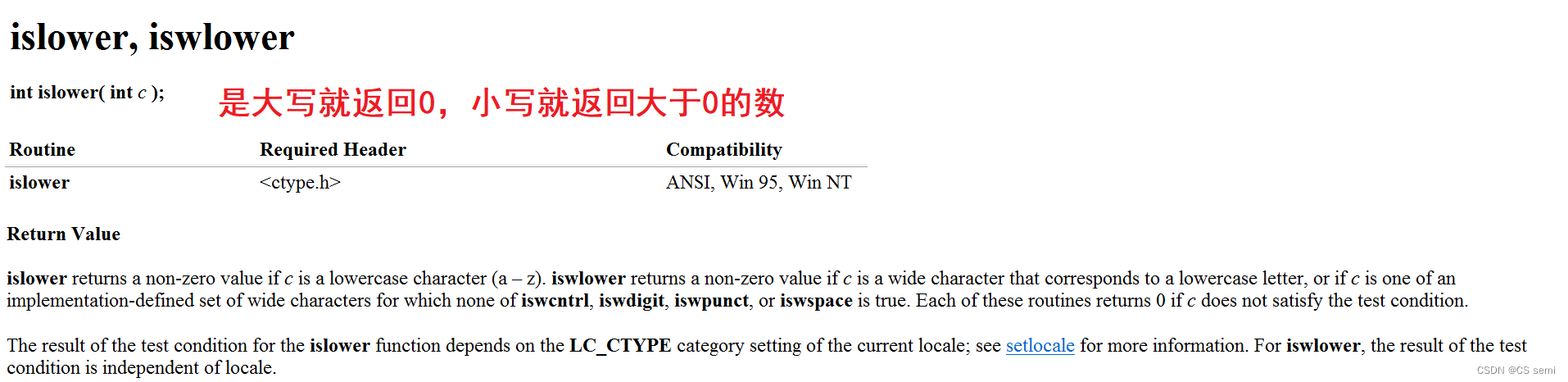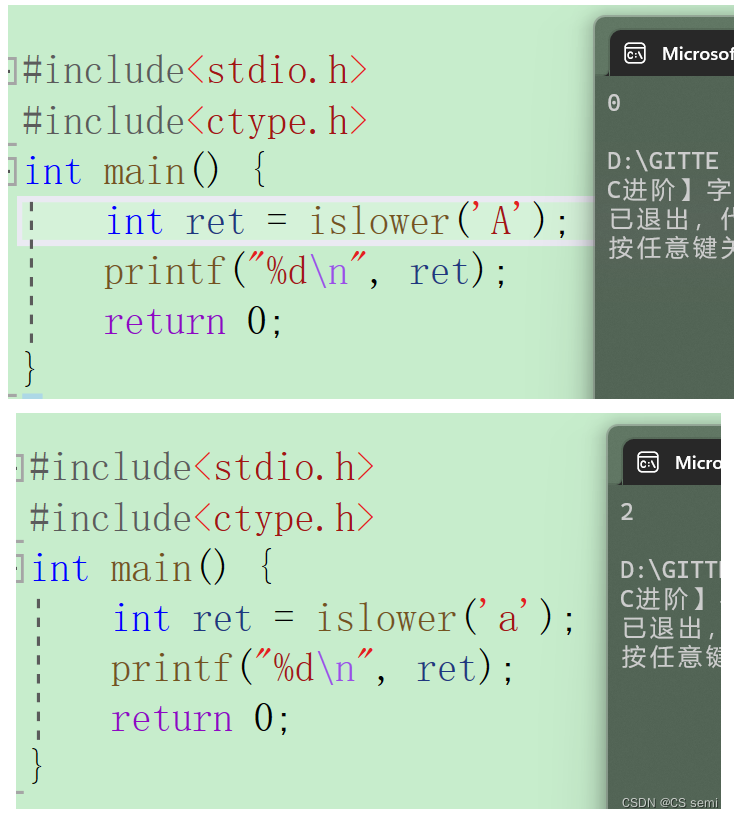# 十二、字符转换

int tolower ( int c );大写字母转小写
int toupper ( int c );小写字母转大写

``````#include<stdio.h>
#include <ctype.h>
int main(){
int i = 0;
char str[] = "Test String.\n";
char c;
while (str[i]){
c = str[i];
if (isupper(c))
c = tolower(c);
putchar(c);
i++;
}
return 0;
}
``````

# 总结

 阿里云国际，腾讯云国际，AWS 免费开户实名账号 代冲值 优惠多多 微信号：monov8 飞机：@monov8